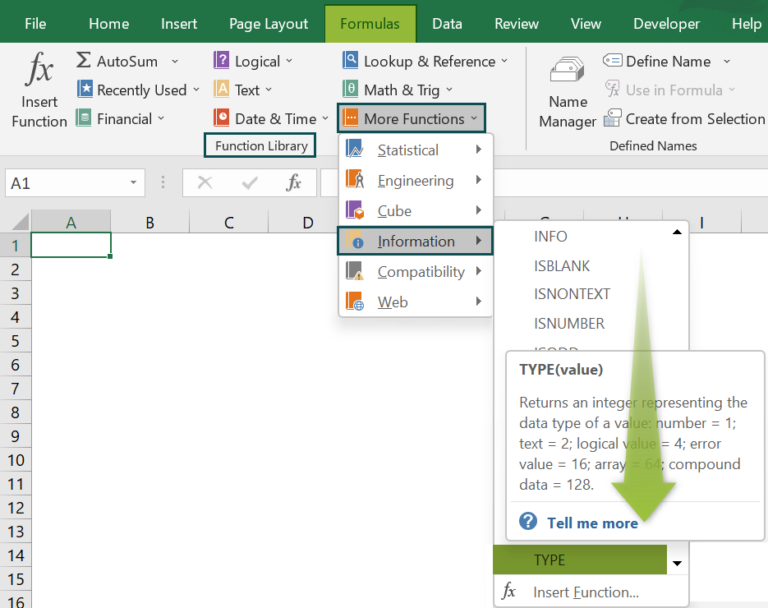# TYPE Function ExcelArticle byExcelMojo Team## What Is TYPE Function In Excel?

The TYPE function Excel returns the numeric value/code that represents the type of value. If the cell value is a formula, then the displayed result’s type value will be returned, as the function only returns the cell values data type of a value. The TYPE Function in Excel is an inbuilt function, so we can insert the formula from the “Function Library” or enter it directly in the worksheet.

The table below shows the type of cell values and the numeric code, which is the return value.

For example, we will apply the TYPE function to calculate the value of the given data.

Select cell B2, enter the formula =TYPE(A2), and press the “Enter” key.

The result is “2”, as shown below.

###### Key Takeaways
• The TYPE function Excel returns the value of the data entered in the cells based on the preset values as follows:
• 1 for numeric value.
• 2 for Textual value.
• 4 for Logical Value [True or False].
• 16 for Error Value.
• 64 for Array Value.
• The TYPE function is useful when using formulas that accept different data types as arguments for input data value. The function first checks for the data type of the result of the formula and then returns its related value.
• The date values must be entered in the Excel recognized format, or else the function will just recognize it as a number, and return the numeric text value.

### TYPE() Excel Formula

The syntax of the TYPE Excel formula is,

The argument of the TYPE Excel formula is,

• value: It is a mandatory argument. It is the cell value to check for the type value.

### How To Use TYPE Excel Function?

We can use the TYPE Excel Function in 2 ways, namely,

1. Access from the Excel ribbon.
2. Enter in the worksheet manually.

#### Method #1 – Access from the Excel ribbon

Choose an empty cell for the output → select the “Formulas” tab → go to the “Function Library” group → click the “More Functions” option drop-down → click the “Information” option right arrow → select the “TYPE” function, as shown below.

The “Function Arguments” window appears. Enter the argument “value” field → click “OK”, as shown below.

#### Method #2 -Enter in the worksheet manually

1. Select an empty cell for the output.
2. Type =TYPE( in the selected cell. [Alternatively, type =T or =TY and double-click the TYPE function from the list of suggestions shown by Excel.
3. Enter the argument as cell values or cell references in excel and close the brackets.
4. Press the “Enter” key.

We will calculate the given value using TYPE Function Excel.

In the table, the data is,

• Column A contains the Data.
• Column B displays the Output.

The steps to evaluate the data using TYPE Formula are,

1. Select cell B2, enter the formula =TYPE(A2), and press the “Enter” key. The result is “2”, as shown below.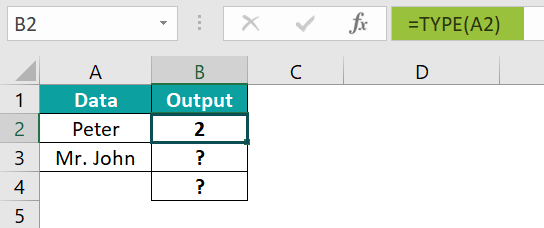2. Drag the formula from cell B2 to B4 using the fill handle.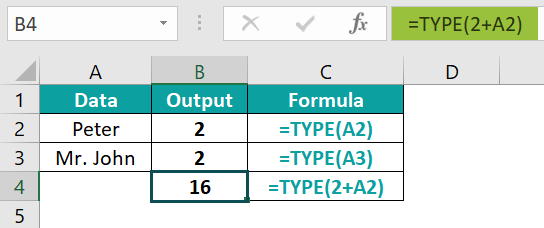The output is shown above. Column C is for our reference.

### Examples

We will understand some advanced scenarios using the TYPE function examples.

#### Example #1

We will calculate the given value using the TYPE Function.

In the table, the data is,

• Column A contains the Data.
• Column B displays the Output.

The steps to evaluate the data using the TYPE formula are,

• Step 1: Select cell B2, enter the formula =TYPE(A2), and press the “Enter” key. The result is “1”, as shown below.
• Step 2: Drag the formula from cell B2 to B6 using the fill handle in excel. The output is shown below.

#### Example #2

We will calculate the value of the given errors as data using the TYPE function.

In the table, the data is,

• Column A contains the Data.
• Column B displays the Output.

The steps to evaluate the data using the TYPE formula are,

• Step 1: Select cell B2, enter the formula =TYPE(A2), and press the “Enter” key. The result is “16”, as shown below.
• Step 2: Drag the formula from cell B2 to B5 using the fill handle.

The output is shown below. The type value for the errors is 16.

#### Example #3

We will calculate the given array values using the TYPE function.

In the table, the data is,

• Column A contains the Data.
• Column B displays the Output.

The procedure to evaluate the data using the TYPE formula is,

Select cell B2, enter the formula =TYPE({1,2,3}), and press the “Enter” key.

The result is “64”, as shown above.

#### Example #4

We will calculate the value of the given data as an error value using the TYPE function.

In the table, the data is,

• Column A contains the Data.
• Column B displays the Output.

The procedure to evaluate the data using the TYPE formulais,

Select cell B2, enter the formula =2+A2, and press the “Enter” key.

The result is a “#VALUE!” error, as shown below.

### Important Things To Note

• If one of the cell values is blank, then the output will return as 1, by default, which is actually the TYPE return value of the date.
• If there is an alphanumeric value like xyz10, then the return value will be 2, i.e., the function will consider it as textual data.
• If there are combinations of text with special characters like abc*&(), the function will still consider it as textual value.

1. What does the TYPE function Excel do?

The TYPE function returns a numeric code representing the data’s TYPE.
The syntax of the TYPE function is =TYPE(value)

2. How does the TYPE function Excel work?

The TYPE function Excel works as follows:

1. Select an empty cell for the output.
2. Type =TYPE( in the selected cell. [Alternatively, type =T or =TY and double-click the TYPE function from the list of suggestions shown by Excel.
3. Enter the argument as cell values or cell references and close the brackets.
4. Press the “Enter” key.

For example, the below image depicts the blank cell, and we will calculate the value of the given blank cell using the TYPE function.

In the table, the data is,
Column A contains the Data.
Column B displays the Output.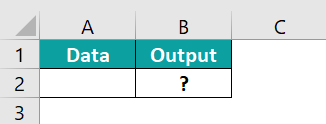The procedure to evaluate the data using the TYPE formula is,

Select cell B2, enter the formula =TYPE(A2), and press the “Enter” key.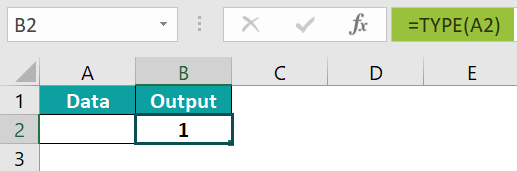The result is “1”, as shown above.

3. Where is the TYPE function in Excel?

The TYPE function is inserted as follows:

Choose an empty cell for the output → select the “Formulas” tab → go to the “Function Library” group → click the “More Functions” option drop-down → click the “Information” option right arrow → select the “TYPE” function, as shown below.